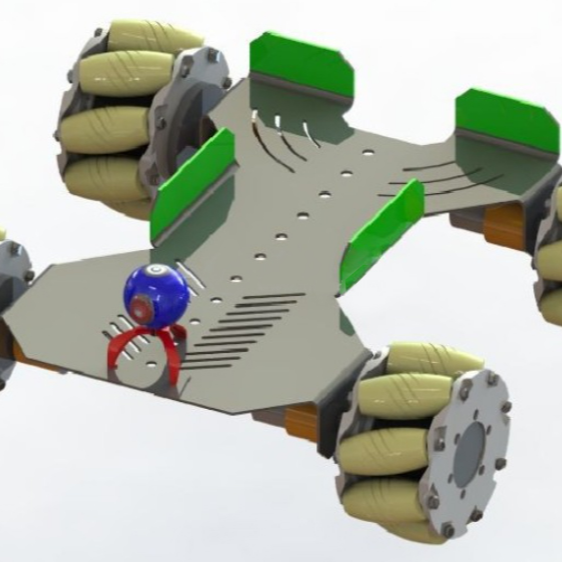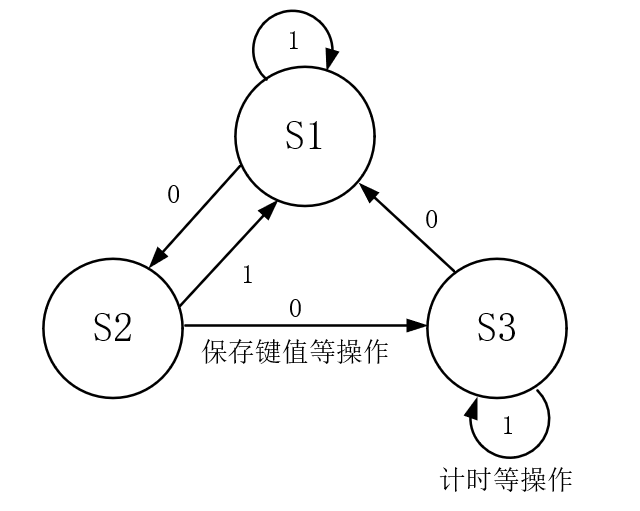# Nebula Pi 开发板应用（一）—利用状态机的思想实现按键检测ii11nnocent 1 年前
# 状态机 # cpu时间

（1）按键未按下的初始状态，设定为 S1
（2）确认有按键按下的状态，设定为 S2
（3）按键按下后释放的状态，设定为 S3KeyState.h:

``````#ifndef _KEYSTATE_H
#define _KEYSTATE_H

#include "reg52.h"

void Timer0_Init(void);
extern unsigned char Times;				//按下的次数
extern bit Flag;					//1s长按标志位

#endif
``````

KeyState.c:

``````#include "KeyState.h"

// 位定义 Key1 按键
sbit Key1 = P1 ^0;
// 保存按键的返回值
unsigned char KeyReturn;
unsigned char Times;
bit Flag;

/****宏定义按键的初始、按下和抬起状态****/
#define Key_State_Init    0
#define Key_State_Down    1
#define Key_State_Up    2

// 按键的状态值，初始值为0
static char KeyState = 0;
// 保存按键的电平值
unsigned char KeyPress;
// 保存P1.0口电平
KeyPress = Key1;

switch (KeyState) {
// 初始状态
case Key_State_Init:
// 读取 IO 电平
if (!KeyPress) {
// 按键返回值为0
KeyReturn = 0;
// 按键的状态转为按键按下的状态
KeyState = Key_State_Down;
}
break;
// 初始状态按下后转为按下状态
case Key_State_Down:
// 读取IO电平确认按键是否真的被按下
if (!KeyPress) {
// 按键返回值为1
KeyReturn = 1;
// 按键的状态转为按键释放的状态
KeyState = Key_State_Up;
} else {
// 如果IO电平为0，证明按键已抬起，回到初始状态
KeyState = Key_State_Init;
}
break;
// 按下以后转为抬起状态
case Key_State_Up:
// 如果按键一直按下，即没有抬起
if (!KeyPress) {
// 只要一直按下按键，Times就会10ms增加1
Times++;
// Times=100时，1s定时时间到，标志位取反并且Times值清零
if (Times == 100) {
Flag = ~Flag;
Times = 0;
}
}
// 如果按键抬起了
if (KeyPress) {
// 按键返回值为 2
KeyReturn = 2;
// 读取 IO 电平，如果是 1，证明按键已释放，回到初始状态
KeyState = Key_State_Init;
}
break;
}
return KeyReturn;
}

void Timer0_Init(void) {
TMOD = 0x01;
TH0 = 0xDC;
// 10ms 定时初值
TL0 = 0x00;
// 开定时器 0 中断
ET0 = 1;
// 开总中断
EA = 1;
// 打开定时器 0
TR0 = 1;
}

/****在中断服务函数中调用按键读取函数，然后主函数中判断键值****/
``````

main.c:

``````#include "reg52.h"
#include "KeyState.h"

sbit Led = P1^7;
sbit Led2 = P1^6;

unsigned char KeyNumber = 0;	//按键值保存变量

void main(void)
{
Timer0_Init();
while(1)
{
if( KeyNumber == 1 )
{
Led = 0;
}
if( KeyNumber == 2 )
{
Led = 1;
}
if( Flag == 1 )
{
Led2 = 0;
}
else	Led2 = 1;
}
}

void Timer0(void) interrupt 1
{
TH0	= 0xDC;
TL0	= 0x00;		//重新赋初值